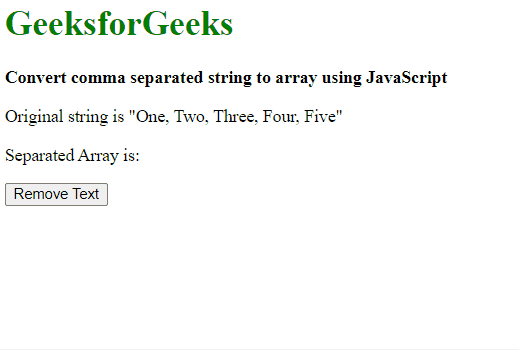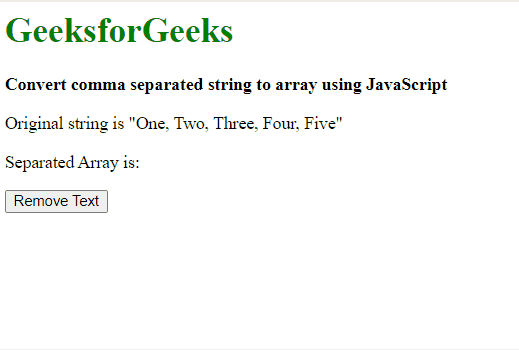Open in App
Not now

# Convert comma separated string to array using JavaScript

• Last Updated : 20 Dec, 2022

A comma-separated string can be converted to an array by 2 approaches:

Method 1: Using JavaScript split() method.

The split() method is used to split a string on the basis of a separator. This separator could be defined as a comma to separate the string whenever a comma is encountered. This method returns an array of strings that are separated.

Syntax:

`string.split(', ')`

Example: In this example, we will be using the split() method to convert a comma-separated string to an array using Javascript.

## html

 `<``body``>` `    ``<``h1` `style``=``"color: green"``>` `        ``GeeksforGeeks` `    ``` `    ``<``b``>Convert comma separated string` `        ``to array using JavaScript` `    ``<``p``>Original string is` `        ``"One, Two, Three, Four, Five"` `    ``<``p``>` `        ``Separated Array is: <``span` `class``=``"output"``>` `    ```   `    ``<``button` `onclick``=``"separateString()"``>` `        ``Remove Text` `    ``` `    ``<``script` `type``=``"text/javascript"``>` `        ``function separateString() {` `            ``originalString = "One, Two, Three, Four, Five";` `            ``separatedArray = originalString.split(', ');` `        `  `            ``console.log(separatedArray);` `            ``document.querySelector('.output').textContent =` `            ``separatedArray;` `        ``}` `    ``` ``

Output:Convert comma separated string to array

Method 2: Iterating through the array keeping track of any comma encountered and creating a new array with the separated strings.

This approach involves iterating through each character in the string and checking for the comma. A variable previousIndex is defined which keeps the track of the first character of the next string. The slice method is then used to remove the portion of the string between the previous index and the current location of the comma found. This string is then pushed onto a new array. This process is then repeated for the whole length of the string. The final array contains all the separated strings.

Syntax:

## javascript

 `originalString = "One, Two, Three, Four, Five";` `separatedArray = [];`   `// index of end of the last string` `let previousIndex = 0;`   `for``(i = 0; i < originalString.length; i++) {`   `// check the character for a comma` `if` `(originalString[i] == ``', '``) {`   `// split the string from the last index` `// to the comma` `separated = originalString.slice(previousIndex, i);` `separatedArray.push(separated);`   `// update the index of the last string` `previousIndex = i + 1;` `}` `}`   `// push the last string into the array` `separatedArray.push(originalString.slice(previousIndex, i));`

Example: In this example, we will be converting a comma-separated value to a Javascript string.

## html

 `<``body``>` `    ``<``h1` `style``=``"color: green"``>` `        ``GeeksforGeeks` `    ``` `    ``<``b``>Convert comma separated` `        ``string to array using JavaScript` `    ``<``p``>Original string is` `        ``"One, Two, Three, Four, Five"` `    ``<``p``>` `        ``Separated Array is: <``span` `class``=``"output"``>` `    ```   `    ``<``button` `onclick``=``"separateString()"``>` `        ``Remove Text` `    ``` `    ``<``script` `type``=``"text/javascript"``>` `        ``function separateString() {` `            `  `            ``originalString =` `            ``"One, Two, Three, Four, Five";` `            ``separatedArray = [];` `        `  `            ``// index of end of the last string` `            ``let previousIndex = 0;` `        `  `            ``for (i = 0; i < ``originalString.length``; i++) {` `        `  `                ``// check the character for a comma` `                ``if (originalString[i] == ', ') {` `        `  `                    ``// split the string from the last index` `                    ``// to the comma` `                    ``separated` `=` `                    ``originalString``.slice(previousIndex, i);` `                    ``separatedArray.push(separated);` `        `  `                    ``// update the index of the last string` `                    ``previousIndex` `= i + 1;` `                ``}` `            ``}` `        `  `            ``// push the last string into the array` `            ``separatedArray.push(` `            ``originalString.slice(previousIndex, i));` `        `  `            ``console.log(separatedArray);` `            ``document.querySelector(` `            ``'.output')``.textContent` `= ``separatedArray``;` `        ``}` `    ``` ``

Output:Convert comma separated string to array

My Personal Notes arrow_drop_up
Related Articles